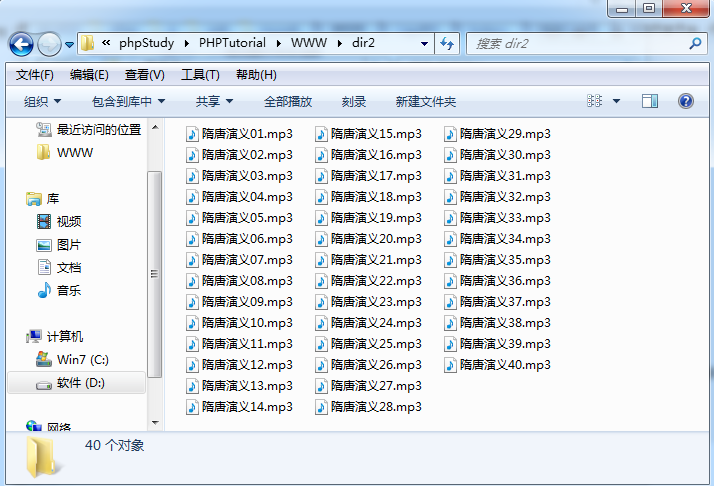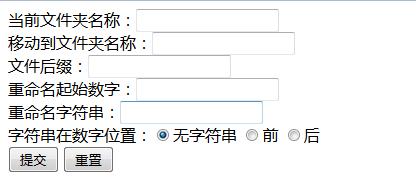php文件夹操作：读取文件夹、批量重命名文件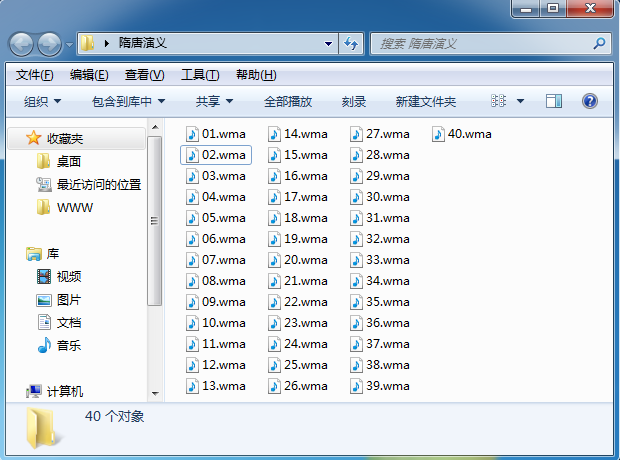<?php
$dir1 = './dir1/';//读取文件夹名称 （重命名为dir1避免中文报错，移动到与要执行的php文件同级目录）$filearr = scandir($dir1);//读取文件夹 print_r($filearr);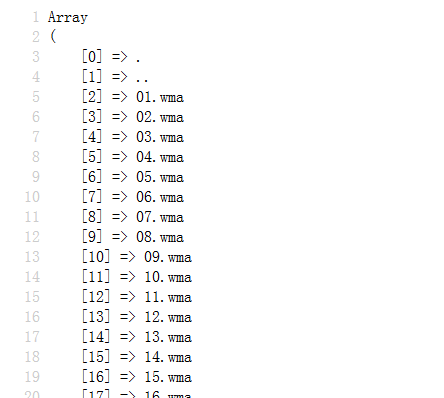<?php
$dir1 = './dir1/';//读取文件夹名称（避免使用中文）$filearr = scandir($dir1);//读取文件夹 unset($filearr);//去除多余的元素
unset($filearr);$filearr = array_values($filearr);//使数组从0开始，以1递增 print_r($filearr);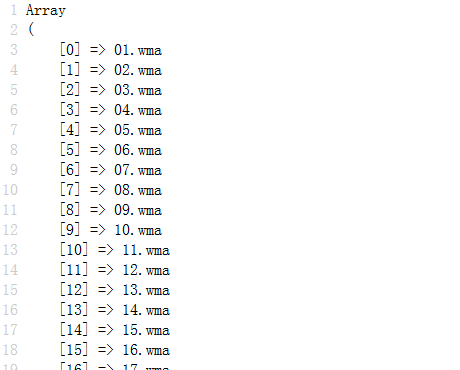<?php
$dir1 = './dir1/';//读取文件夹名称（避免使用中文）$dir2 = './dir2/';//移动到文件夹名称（避免使用中文）
$filearr = scandir($dir1);//读取文件夹
unset($filearr);//去除多余的元素 unset($filearr);
$filearr = array_values($filearr);//使数组从0开始，以1递增
$filecount = count($filearr);//文件数量
$onenum = 1;//起始编号$maxnum = $onenum +$filecount;//最大循环次数
$title = 'styy';//文件标题$houzhui = '.mp3';//文件后缀
for ($i =$onenum; $i <=$maxnum; $i++) { for ($j=0; $j <$filecount ; $j++) { if($i<10){
$i = '0'.$i;
}
rename($dir1.$filearr[$j],$dir2.$title.$i.$houzhui);$i++;
}
}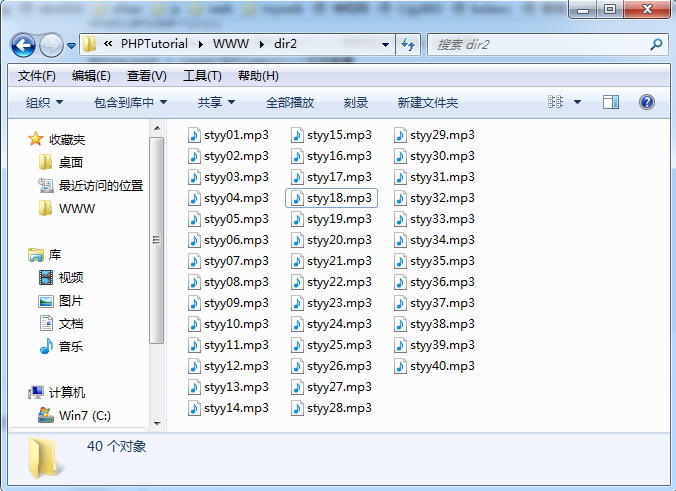<?php
$dir1 = './dir1/';//读取文件夹名称$dir2 = './dir2/';//移动到文件夹名称
$filearr = scandir($dir1);//读取文件夹
unset($filearr);//去除多余的元素 unset($filearr);
$filearr = array_values($filearr);//使数组从0开始，以1递增
$filecount = count($filearr);//文件数量
$onenum = 1;//起始编号$maxnum = $onenum +$filecount;//最大循环次数
$title = '隋唐演义';//文件标题$houzhui = '.mp3';//文件后缀
for ($i =$onenum; $i <=$maxnum; $i++) { for ($j=0; $j <$filecount ; $j++) { if($i<10){
$i = '0'.$i;
}
$filename =$title.$i.$houzhui;
rename($dir1.$filearr[$j],$dir2.iconv("utf-8","gb2312",$filename));//设置字符集$i++;
}
}